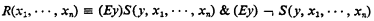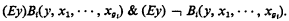Home

# A reduction theorem for predicate logic

## Extract

It is well known that in prepositional logic not every propositional connective is definable in terms of equivalence nor indeed in terms of equivalence together with negation. It is the purpose of this note to show that in a certain sense this is, however, possible within predicate logic.

Let F1 be the class of predicate logical formulae containing only the logical connectives ¬ (not), ∨ (or), E (exists), and F2 the class of predicate logical formulae containing only the logical connectives ≡ (if and only if) and E.

Our object will be to find a method for reducing any formulae ϕ of F1 to a formula ψ of F2 such that ϕ is valid if and only if ψ is valid.

Note first that for every domain D containing at least two individuals we can for every n-place relation R defined over D find an n + 1-place relation S defined over D such that for all x1, …, xnD,holds. For instance, let S(y, x1, …, xn) be the relation y = x1 & R(x1, …, xn).

Now let ϕ be an arbitrary formula of F1 and A1, …, A3 a complete list of predicate letters occurring in ϕ of degree g1, …, gi, respectively. Let ϕ′ be the formula obtained from ϕ by replacing every prime formula Ai(x1, …, xgi) byThen it follows immediately from the previous observation that (a) ϕ is satisfiable in D if and only if ϕ′ is satisfiable in D.

# A reduction theorem for predicate logic

## Metrics

### Full text viewsFull text views reflects the number of PDF downloads, PDFs sent to Google Drive, Dropbox and Kindle and HTML full text views.

Total number of HTML views: 0
Total number of PDF views: 0 *Loading metrics...

### Abstract viewsAbstract views reflect the number of visits to the article landing page.

Total abstract views: 0 *Loading metrics...

* Views captured on Cambridge Core between <date>. This data will be updated every 24 hours.

Usage data cannot currently be displayed.